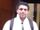## Extracting values from PROC MEANS, use them in a new table to compute .

Hi, I'm looking for a fancy way to solve my problem. Even though the code is doing what I need I am not happy with it.

What I need is to take values from two OUTPUTs obtained by PROC LOGISTIC and PROC MEANS.

How can I assign the mean value obtained from PROC MEANS to a new variable? In this case, I have two means, one where the class is 1 and the other when the class is 0. Therefore, I need to create a variable called mean_y_1 and  mean_y_0. I assigned those values by hand, how can I do using functions?.  Check the code below.

Thank you.

``````DATA ORIGINAL;
INPUT ID Y CLASS;
CARDS;
1 12000 1
2 0 0
3 0.6 0
4 0.7 1
5 19 0
;
RUN;

PROC LOGISTIC DATA = ORIGINAL PLOT = NONE OUTEST = LOGIT_ESTIMATESS;
MODEL  CLASS(EVENT = '1')= Y /; RUN;

PROC MEANS DATA = ORIGINAL n MEAN STD MAXDEC = 4;
CLASS CLASS;  VAR ID; OUTPUT OUT= ORIGINAL_MEANS MEAN= MEAN_Y; RUN;

/* TAKING VALUES FROM LOGIT_ESTIMATES AND ORIGINAL_MEANS. BOTH TABLES ARE THE OUTPUT OF PROC LOGISTIC AND PROC MEANS */

DATA ORIGINAL_ANALYSIS; DROP _FREQ_ _TYPE_ ; SET LOGIT_ESTIMATESS;
/* ASSIGNING THE MEAN VALUE BY CLASS */
MEAN_Y_1 = 3.3333;
MEAN_Y_0 = 2.5000;

/* WHEN CLASS = 1 */
STEP1_1 = INTERCEPT + (Y * MEAN_Y_1) ;
/* WHEN CLASS = 0 */
STEP1_0 = INTERCEPT + (Y * MEAN_Y_0) ;

FORMAT STEP1_1  STEP1_0 COMMA32.31;
LABEL STEP1_1 = 'AVERAGE METHOD PROBABILITY OF CLASS = 1';
LABEL STEP1_0 = 'AVERAGE METHOD PROBABILITY OF CLASS = 1';
RUN;
PROC PRINT DATA = ORIGINAL_ANALYSIS LABEL; FORMAT STEP1_1 STEP1_0 COMMA10.4 ;VAR STEP1_1 STEP1_0 ; RUN;``````

1 ACCEPTED SOLUTION

Accepted Solutionsnovinosrin
Tourmaline | Level 20

## Re: Extracting values from PROC MEANS, use them in a new table to compute .

a fix

``````
PROC MEANS DATA = ORIGINAL n MEAN STD MAXDEC = 4 nway;
CLASS CLASS;  VAR ID; OUTPUT OUT= ORIGINAL_MEANS MEAN= MEAN_Y; RUN;

DATA ORIGINAL_ANALYSIS;  SET LOGIT_ESTIMATESS;
if _n_=1 then do;
set ORIGINAL_MEANS(firstobs=1 keep=mean_y rename=(mean_y=  MEAN_Y_0));
set ORIGINAL_MEANS(firstobs=2 keep=mean_y rename=(mean_y=  MEAN_Y_1));
end;
/* ASSIGNING THE MEAN VALUE BY CLASS */
/*	MEAN_Y_1 = 3.3333;*/
/* 	MEAN_Y_0 = 2.5000;*/

/* WHEN CLASS = 1 */
STEP1_1 = INTERCEPT + (Y * MEAN_Y_1) ;
/* WHEN CLASS = 0 */
STEP1_0 = INTERCEPT + (Y * MEAN_Y_0) ;

FORMAT STEP1_1  STEP1_0 COMMA32.31;
LABEL STEP1_1 = 'AVERAGE METHOD PROBABILITY OF CLASS = 1';
LABEL STEP1_0 = 'AVERAGE METHOD PROBABILITY OF CLASS = 1';
RUN;``````
2 REPLIES 2novinosrin
Tourmaline | Level 20

## Re: Extracting values from PROC MEANS, use them in a new table to compute .

a fix

``````
PROC MEANS DATA = ORIGINAL n MEAN STD MAXDEC = 4 nway;
CLASS CLASS;  VAR ID; OUTPUT OUT= ORIGINAL_MEANS MEAN= MEAN_Y; RUN;

DATA ORIGINAL_ANALYSIS;  SET LOGIT_ESTIMATESS;
if _n_=1 then do;
set ORIGINAL_MEANS(firstobs=1 keep=mean_y rename=(mean_y=  MEAN_Y_0));
set ORIGINAL_MEANS(firstobs=2 keep=mean_y rename=(mean_y=  MEAN_Y_1));
end;
/* ASSIGNING THE MEAN VALUE BY CLASS */
/*	MEAN_Y_1 = 3.3333;*/
/* 	MEAN_Y_0 = 2.5000;*/

/* WHEN CLASS = 1 */
STEP1_1 = INTERCEPT + (Y * MEAN_Y_1) ;
/* WHEN CLASS = 0 */
STEP1_0 = INTERCEPT + (Y * MEAN_Y_0) ;

FORMAT STEP1_1  STEP1_0 COMMA32.31;
LABEL STEP1_1 = 'AVERAGE METHOD PROBABILITY OF CLASS = 1';
LABEL STEP1_0 = 'AVERAGE METHOD PROBABILITY OF CLASS = 1';
RUN;``````

## Re: Extracting values from PROC MEANS, use them in a new table to compute .

Thanks that's what I was looking.

Works perfectly.

Cheers

Discussion stats
• 2 replies
• 514 views
• 3 likes
• 2 in conversation